SBOS823 December   2018

PRODUCTION DATA.

1. Features
2. Applications
3. Description
1.     Device Images
4. Revision History
5. Pin Configuration and Functions
6. Specifications
7. Detailed Description
1. 7.1 Overview
2. 7.2 Functional Block Diagram
3. 7.3 Feature Description
4. 7.4 Device Functional Modes
8. Application and Implementation
1. 8.1 Application Information
2. 8.2 Typical Application
3. 8.3 System Examples
9. Power Supply Recommendations
10. 10Layout
11. 11Device and Documentation Support
12. 12Mechanical, Packaging, and Orderable Information

• D|8
• DGK|8
• DGK|8

### 6.8 Typical Characteristics

At TA = 25°C, VS = 5 V, RL = 10 kΩ connected to VS / 2, and VCM = VOUT = VS / 2, unless otherwise noted.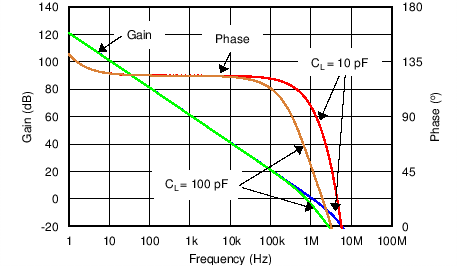Figure 1. Open-Loop Gain and Phase vs FrequencyFigure 3. Quiescent Current vs SupplyFigure 5. Offset Voltage Production Distribution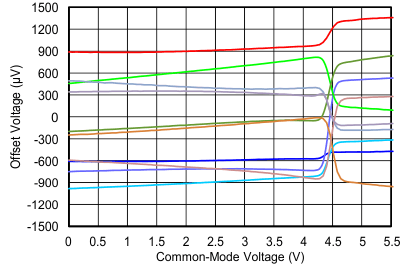Figure 7. Offset Voltage vs Common-Mode Voltage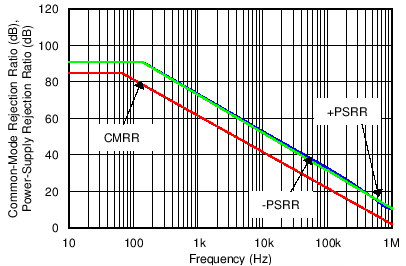Figure 9. CMRR and PSRR vs Frequency
(Referred-to-Input)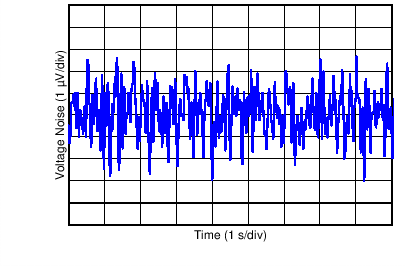Figure 11. 0.1-Hz to 10-Hz Input Voltage Noise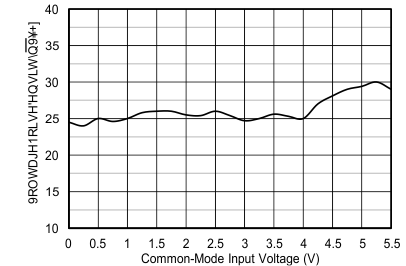Figure 13. Voltage Noise vs Common-Mode Voltage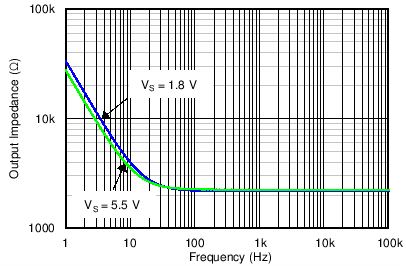Figure 15. Open-Loop Output Impedance vs Frequency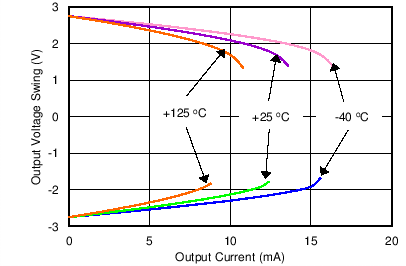Figure 17. Output Voltage Swing vs Output Current (Over Temperature)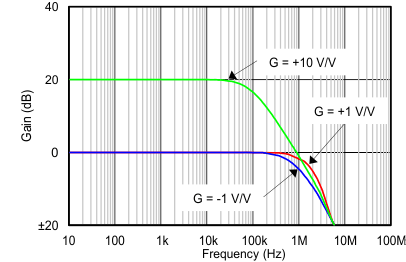Figure 19. Closed-Loop Gain vs Frequency
(Maximum Supply)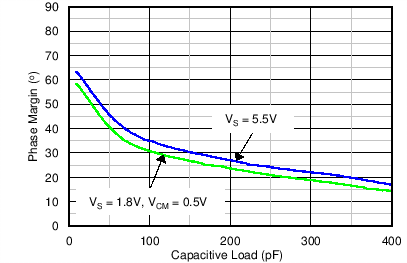Figure 21. Phase Margin vs Capacitive Load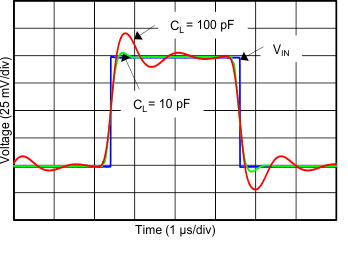Figure 23. Small-Signal Pulse Response
(Maximum Supply)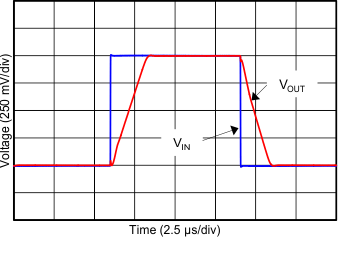Figure 25. Large-Signal Pulse Response
(Maximum Supply)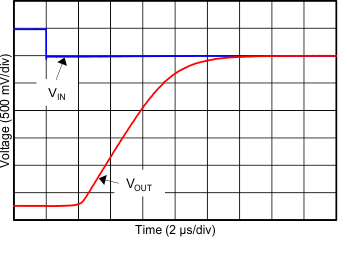Figure 27. Negative Overload Recovery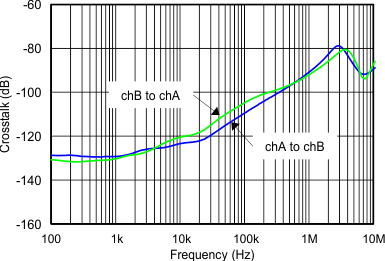Figure 29. Channel Separation vs Frequency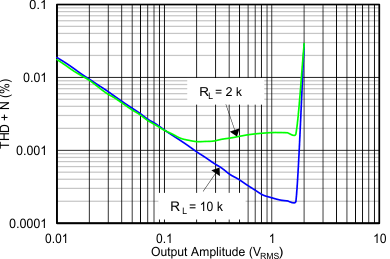Figure 31. THD+N vs Output Amplitude
(Maximum Supply)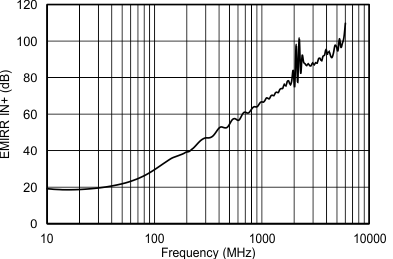Figure 33. EMIRR IN+ vs Frequency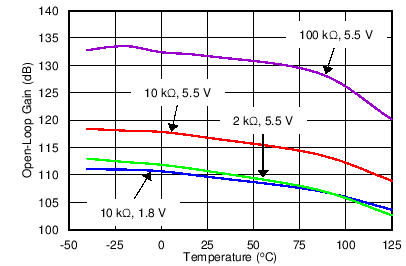Figure 2. Open-Loop Gain vs TemperatureFigure 4. Quiescent Current vs Temperature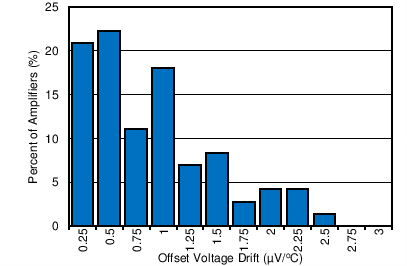Figure 6. Offset Voltage Drift Distribution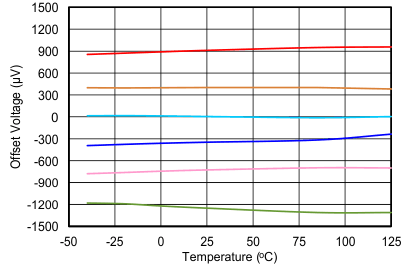Figure 8. Offset Voltage vs Temperature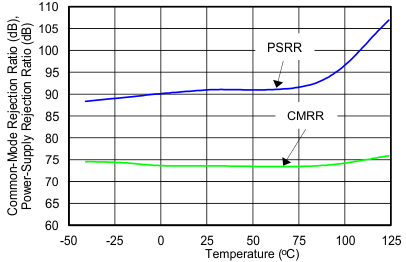Figure 10. CMRR and PSRR vs Temperature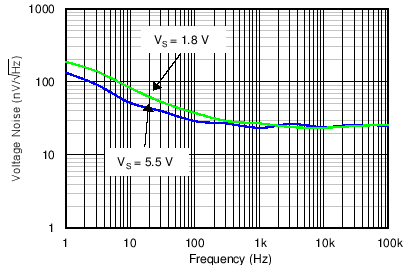Figure 12. Input Voltage Noise Spectral Density vs Frequency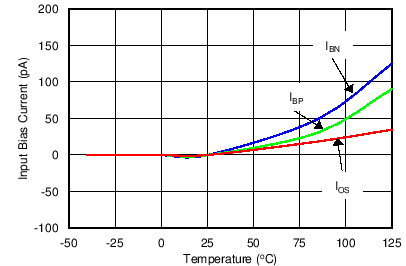Figure 14. Input Bias and Offset Current vs Temperature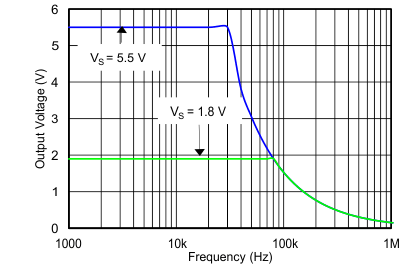Figure 16. Maximum Output Voltage vs Frequency and Supply Voltage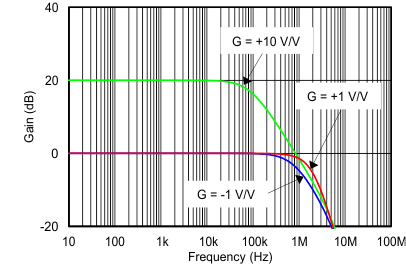Figure 18. Closed-Loop Gain vs Frequency (Minimum Supply)Figure 20. Small-Signal Overshoot vs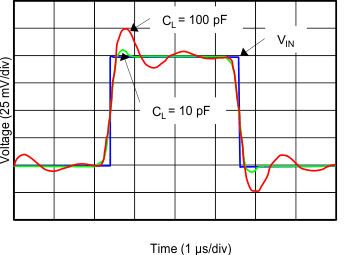Figure 22. Small-Signal Pulse Response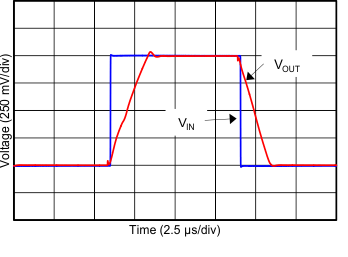Figure 24. Large-Signal Pulse Response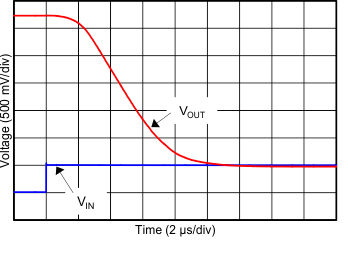Figure 26. Positive Overload Recovery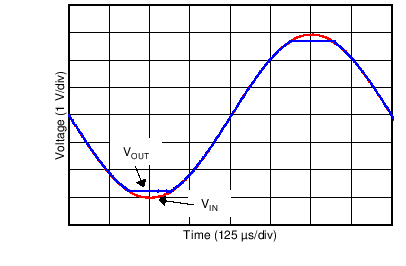Figure 28. No Phase Reversal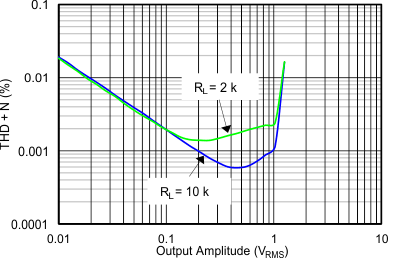Figure 30. THD+N vs Output Amplitude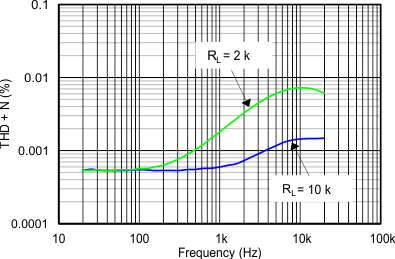Figure 32. THD+N vs Frequency